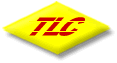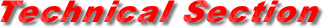xx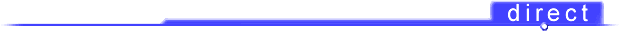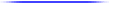Inverters 12v dc to 240v ac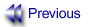Index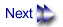CAUTION! INVERTER's must be connected only to batteries with the corect output voltage
The 12 volt INVERTER must be connected only to a 12 volt battery system
The 24 volt INVERTER must be connected only to a 24 volt battery system.

Battery Sizing
There are a number of different standards for rating battery energy storage capacity. Automotive starting batteries are normally rated by cranking amps. This is not a relevant rating, for continuous use. Deep-cycle batteries are rated either by reserve capacity in minutes or by ampere-hour capacity.

Battery reserve capacity is a measure of how long a battery can deliver a certain amount of current - usually 25 amperes. For instance, a battery with a reserve capacity of 180 minutes can deliver 25 amperes for 180 minutes before it is completely discharged.

Ampere-hour capacity is a measure of how many amperes a battery can deliver for a specified length of time- usually 20 hours. For example, a typical marine or RV battery rated for 100 ampere-hours can deliver 5 amperes for 20 hours (5 amperes x 20 hours = 100 amp-hrs).

Actual battery capacity decreases as discharge current increases. A battery rated at 100 ampere-hours which can deliver 5 amperes for 20 hours, may deliver 20 amperes for only 4 hours, resulting in an actual capacity of 80 ampere-hours. For this reason, it is difficult to compare rated ampere-hour capacity with battery reserve capacity. For example a battery with a reserve capacity of 180 minutes has the following calculated ampere- hour capacity

180 min. Divided by 60 = 3 hr., 3 hr. x 25 amps = 75 amp-hrs

However its actual ampere-hour rating will be closer to 100 ampere-hours because it is rated at the discharge current required to get 20 hours of operation (about 5 amperes).

To determine the battery capacity you require, follow these steps:

STEP 1- For each piece of equipment you will be operating from 2500W INVERTER, determine how many watts it consumes. This can normally be found on a label on the product. If only the current draw is given, multiply the current draw by 115 (or 225) to get the power consumption in watts.

STEP 2- For each piece of equipment you will be operating from 2500W INVERTER, estimate how many hours it will operate between battery charging cycles.

STEP 3 - Calculate total watt-hours of energy consumption, total hours running time, and average power consumption as in the following example.

STEP 4 - Using the chart below (Table 3 ), find the battery size that will give you the required operating time at the calculated average power consumption. For instance, from the example below, the required operating time is 6 hours and the average power consumption is 387 watts. From the chart, the smallest battery size which will give more than 6 hours of operation at a power level between 300 and 400 watts are two 400 amp-hr. batteries in parallel, which offers between 10 and 12 hours of operating time.